Kristen Beck

•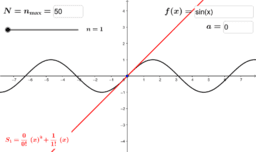Taylor Polynomials

Activity

Kristen Beck

•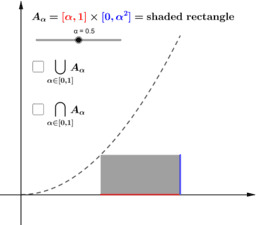A product of real-indexed sets

Activity

Kristen Beck

•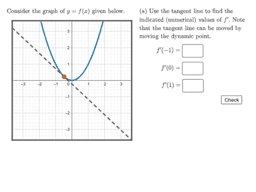The values and graph of a derivative

Activity

Kristen Beck

•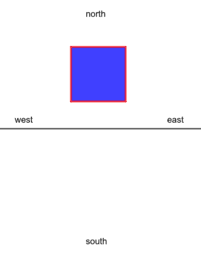A Square passing through Lineland

Activity

Kristen Beck

•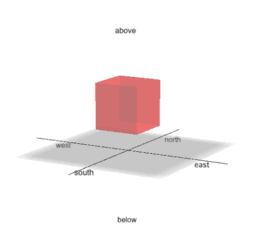A Cube passing through Flatland

Activity

Kristen Beck

•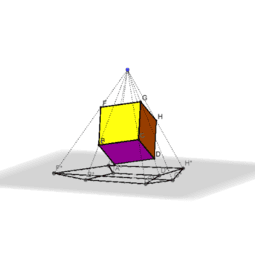Projections of a rotating 3-cube

Activity

Kristen Beck

•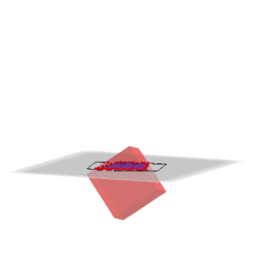Cross-sections of a 3-cube inside its projection

Activity

Kristen Beck

•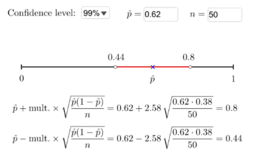Confidence Interval for a Single Proportion

Activity

Kristen Beck

•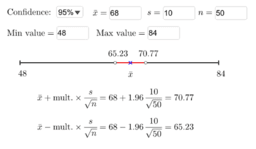Confidence Interval for a Single Mean

Activity

Kristen Beck

•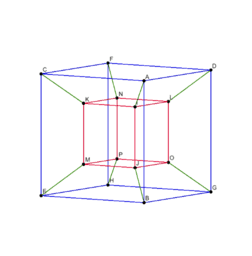Perspective Projection of a 4-Cube

Activity

Kristen Beck

•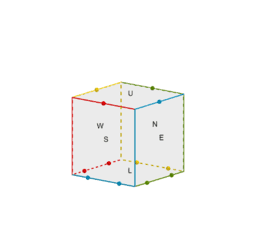Faces and Edges of a 3-Cube

Activity

Kristen Beck

•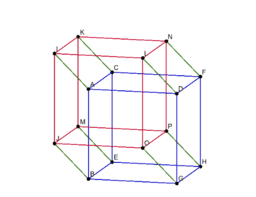Parallel Projection of a 4-Cube

Activity

Kristen Beck

•Mobius Strip

Activity

Kristen Beck

•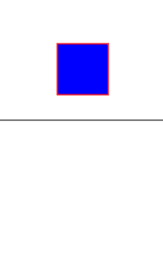Cross-Sections of a 2-Cube

Activity

Kristen Beck

•Cross-Sections of a 3-Cube

Activity

Kristen Beck

•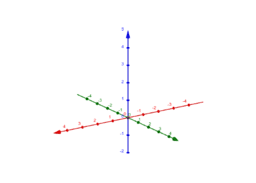Orientation

Activity

Kristen Beck

•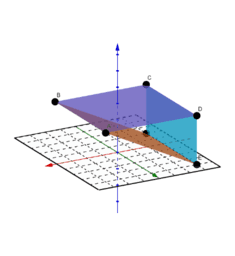Using Rectangular Coordinates

Activity

Kristen Beck

•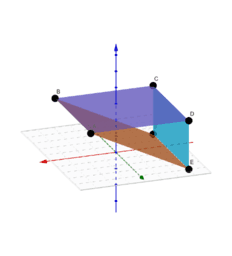Using Rectangular Coordinates

Activity

Kristen Beck

•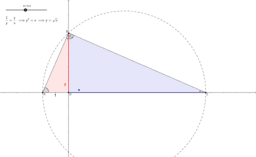Art & Practice of Math

Book

Kristen Beck

•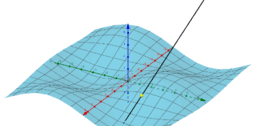Activity

Kristen Beck

•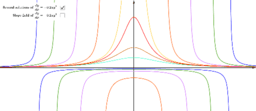Slope Field Plotter

Activity

Kristen Beck

•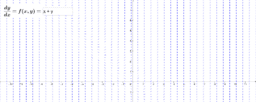Slope Field Plotter

Activity

Kristen Beck

•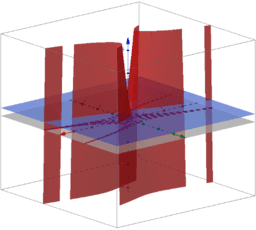Level Curves

Activity

Kristen Beck

•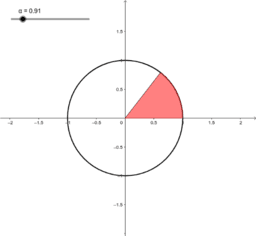Activity

Kristen Beck